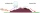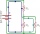# Degrees FahrenheitThe equation above shows how temperature F, measured in degrees Fahrenheit, relates to a temperature C, measured in degrees Celsius. Based on the equation, which of the following must be true?

I. A temperature increase of 1 degree Fahrenheit is equivalent to a temperature increase of 5/9 degree Celsius.
II. A temperature increase of 1 degree Celsius is equivalent to a temperature increase of 1.8 degrees Fahrenheit.
III. A temperature increase of 5/9 degree Fahrenheit is equivalent to a temperature increase of 1 degree Celsius.

A) I only
B) II only
C) III only
D) I and II only

Result

x = (Correct answer is: D)#### Solution:Leave us a comment of example and its solution (i.e. if it is still somewhat unclear...):

Showing 0 comments:Be the first to comment!#### To solve this verbal math problem are needed these knowledge from mathematics:

Need help calculate sum, simplify or multiply fractions? Try our fraction calculator.

## Next similar examples:

1. ImagineImagine that a unit of air at a temperature of 25°C rises up a mountain range that is 3,000 meters high on the windward side and which descends to 1,200 meters on the leeward side, assuming that the air will remain dry what will its temperature when it cro
2. AsymptoteWhat is the vertical asymptote of ?
3. Quadratic function 2Which of the points belong function f:y= 2x2- 3x + 1 : A(-2, 15) B (3,10) C (1,4)
4. Average temperatureThe average temperature from Monday to Sunday was 40.5 and the average temperature from Monday to Saturday was 42.8. What was the temperature on Sunday?
5. Effective and mean voltageA voltage divider consisting of resistors R1 = 103000 Ω and R2 = 197000 Ω is connected to the ideal sine wave voltage source, R2 is connected to a voltmeter which measures the mean voltage and has an internal resistance R3 = 200300 Ω, the measured value is
6. Theorem proveWe want to prove the sentence: If the natural number n is divisible by six, then n is divisible by three. From what assumption we started?
7. Functions f,gFind g(1) if g(x) = 3x - x2 Find f(5) if f(x) = x + 1/2
8. BlocksThere are 9 interactive basic building blocks of an organization. How many two-blocks combinations are there?
9. AverageIf the average(arithmetic mean) of three numbers x,y,z is 50. What is the average of there numbers (3x +10), (3y +10), (3z+10) ?
10. SequenceWrite the first 7 members of an arithmetic sequence: a1=-3, d=6.
11. ChordsHow many 4-tones chords (chord = at the same time sounding different tones) is possible to play within 7 tones?
12. TeamsHow many ways can divide 16 players into two teams of 8 member?
13. LineIt is true that the lines that do not intersect are parallel?
14. Reference angleFind the reference angle of each angle:
15. PIN - codesHow many five-digit PIN - code can we create using the even numbers?
16. ConfectioneryThe village markets have 5 kinds of sweets, one weighs 31 grams. How many different ways a customer can buy 1.519 kg sweets.
17. ExaminationThe class is 21 students. How many ways can choose two to examination?